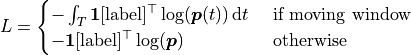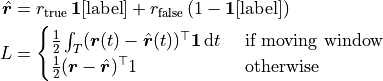# Loss

This module provides some pre-built loss methods to be used with spike-train. Standard PyTorch loss are also compatible.

class lava.lib.dl.slayer.loss.SpikeMax(moving_window=None, mode='probability', reduction='sum')

Spike max (NLL) loss.Note: input is always collapsed in spatial dimension.

Parameters
• moving_window (int) – size of moving window. If not None, assumes label to be specified at every time step. Defaults to None.

• mode (str) – confidence mode. One of ‘probability’|’softmax’. Defaults to ‘probability’.

• reduction (str) – loss reduction method. One of ‘sum’|’mean’. Defaults to ‘sum’.

forward(input, label)

Forward computation of loss.

class lava.lib.dl.slayer.loss.SpikeRate(true_rate, false_rate, moving_window=None, reduction='sum')

Spike rate loss.Note: input is always collapsed in spatial dimension.

Parameters
• true_rate (float) – true spiking rate.

• false_rate (float) – false spiking rate.

• moving_window (int) – size of moving window. If not None, assumes label to be specified at every time step. Defaults to None.

• reduction (str) – loss reduction method. One of ‘sum’|’mean’. Defaults to ‘sum’.

forward(input, label)

Forward computation of loss.

class lava.lib.dl.slayer.loss.SpikeTime(time_constant=5, length=100, filter_order=1, reduction='sum')

Spike-time based loss. It is similar to van Rossum distance between output and desired spike train.Parameters
• time_constant (int) – time constant of low pass filter. Defaults to 5.

• length (int) – length of low pass filter. Defaults to 100.

• filter_order (int) – order of low pass filter. Defaults to 1.

• reduction (str) – mean square reduction. Options are ‘mean’|’sum’. Defaults to ‘sum’.

forward(input, desired)

Forward computation of loss.